# Discussion Forum

## How to calculate the volume of a hole inside an axisymmetric 2D domain (?)

Hi!

I explain the problem.

I have a solid that it deforms over time under the action of loads (elastic+creep).

The solid is a cylinder with a hole with sphere geometry (initial) in the center. With the passage of time the geometry of the sphere will change and also its volume. The problem I have is that the sphere is not a mesh domain, so I am not able to calculate its volume with a double integral and including 2pir (by clicking the postprocessing box), or creating an expression like this 2pir. I am able to calculate the surface of the contour but not the volume.

I solve the temporal evolution of the volume by including nonlinear geometry in the solver. And as an "approximation" to solve the problem I have included a material with properties of "air" in the hole. But I am concerned that as I make the model more complex, this jump between the properties of the cylinder and those of the artificial domain "air" that I have created could give me problems. I prefer being able to calculate the volume directly.

Somebody could help me? I've been looking at the Forum for days and there's a lot of information about axisymmetric 2D volumes but nothing works. I think the problem is the fact that I have to calculate the volume of a hole or external boundary.

Attached two pics.

Best! AS

19 Replies Last Post Jan 25, 2023, 9:54 a.m. EST

Posted: 2 months ago
Updated: 2 months ago

Do you have the coordinates (x,z) of the bounding curve of the data? If so, it seems like you could apply traditional calculus. E.g., https://www.cuemath.com/questions/how-to-find-the-volume-of-a-solid-rotated-around-the-y-axis/ (In your case, you would use z instead of y.)

Posted: 2 months ago
Updated: 2 months ago

Dear Mr. Koslover,

I have applied this method and another variant that is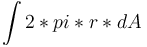, where dA would be dr*dz (area differential). However, neither of the two methods gives a correct result for a contrast test with a sphere of 10 m radius.

I think the problem is that the COMSOL simple integral operator calculates the line integral, that is: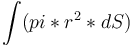, where dS is the differential arc length of the boundary, but I would need it to do the integral: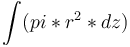where dz is the height differential and I don't know how to do this.

Applying: "Results>Derived values>Line integration" on the boundary shaded in blue of the figure "hole_in_volume_2D_axi" with the "Expression (1)" half the length of the circle is obtained. And if I carry out the same operation, in that same contour but applying the "Expression (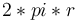)" I obtain the surface of the sphere that encloses the volume that I want to calculate. In the case of a sphere, I could deduce the volume from the surface (as long as the deformation is low). But this does not interest me, I only use the sphere as a test to know that I am doing the calculations correctly, I need the volume to be calculated in a generic way for any type of boundary that generates a hole within the axisymmetric 2D model.

If you can help me I would greatly appreciate it.

Kind Regards, Andres

Posted: 2 months ago
Updated: 2 months ago

Andres,

I think this can be done much simpler. You can mesh the hole domain but keep it outside your physics. You can then use a volume integration to measure the volume.

Cheers Edgar

-------------------
Edgar J. Kaiser
emPhys Physical Technology
www.emphys.com

Posted: 2 months ago

Dear Mr. Kaiser,

I am doing something similar as a "reasonable approximation" ;) . What I do is generate a domain inside the hole, I assign it some properties that make it almost an "absurd" material. A very low modulus of elasticity, a ridiculous Poisson's ratio, etc. Then I mesh the whole problem and ask COMSOL to calculate problem and evaluate the volume (with a double integral) of that domain of "physical nonsense/absurd stuff".

What worries me about this strategy is that the properties of the domain that I am calculating are very different from those of the "auxiliary" material that I am putting into the hole, and as I make the model more complex I am afraid that it will generate problems with the solver... convergence problems, very abrupt jumps in the stress state, etc.

From what I understand from your post, is there a way to make COMSOL mesh a domain but not solve the problem on that domain (?). If so, could you guide me a bit on how to do it? I would really appreciate your help with this issue.

Thank you very much for your attention,

Kind Regards, Andres

Posted: 2 months ago
Updated: 2 months ago

Yes, you can make a mesh even without any physics in the model. You may need to switch off the physics controlled meshing and set up a user controlled mesh. Select the hole domain and apply the mesh you desire.

-------------------
Edgar J. Kaiser
emPhys Physical Technology
www.emphys.com

Posted: 2 months ago

Ok!

Thank you very much!

I will try this ;)

Thank you very much again Mr. Kaiser,

Best, Andres

Posted: 2 months ago
Updated: 1 month ago

Hello Andres,

There is, as you suggested in another thread, an elegant solution based on the Divergence Theorem (DT) that does not require meshing the hole,

Here's how.

volume integral of div(F) = surface integral of (F.n)

where n is the normal vector pointing away from the hole

The trick is to choose F=z*ez

where ez is the base vector in the z direction.

Then div(F)=1 and the l.h.s. of the DT is the volume you want to compute.

The r.h.s. of the DT, accounting for the axisymmetry, is

line integral of 2*pi*z*r*nz

where nz is the z-direction component of the normal vector pointing away from the hole.

Voila!

This approach is implemented in the attached file. You can see that it checks out on a sphere and cylinder.

Notes: 1/ COMSOL's normal vector points away from the computational domain, so into the hole; that's why there is a minus sign in my implemented formula. 2/ I checked off the "compute volume integral" checkbox to take manual control of the axisymmetric integration.

Best,

Jeff

-------------------
Jeff Hiller

Posted: 1 month ago

The same idea using the divergence theorem has been used here:

https://www.comsol.com/blogs/computing-controlling-volume-cavity

-------------------
Henrik Sönnerlind
COMSOL

Posted: 1 week ago

Hello Andres,

There is, as you suggested in another thread, an elegant solution based on the Divergence Theorem (DT) that does not require meshing the hole,

Here's how.

volume integral of div(F) = surface integral of (F.n)

where n is the normal vector pointing away from the hole

The trick is to choose F=z*ez

where ez is the base vector in the z direction.

Then div(F)=1 and the l.h.s. of the DT is the volume you want to compute.

The r.h.s. of the DT, accounting for the axisymmetry, is

line integral of 2*pi*z*r*nz

where nz is the z-direction component of the normal vector pointing away from the hole.

Voila!

This approach is implemented in the attached file. You can see that it checks out on a sphere and cylinder.

Notes: 1/ COMSOL's normal vector points away from the computational domain, so into the hole; that's why there is a minus sign in my implemented formula. 2/ I checked off the "compute volume integral" checkbox to take manual control of the axisymmetric integration.

Best,

Jeff

Thank you very much for your explanation Jeff!

The proposed method works perfectly :)

In choosing the function to apply the DT, a Professor from my University has proposed another function: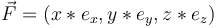.

So the divergence of this function would be: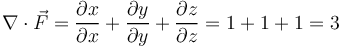.

Due to the properties of the Divergence, this value is invariant even if we change the cartesian coordinate system to cylindrical coordinates, those of an axisymmetric 2D problem. The function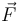, however, changes its expression. Cartesian coordinates are written in terms of cylindrical coordinates: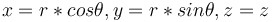;

and the orthonormal vectors in cylindrical coordinates: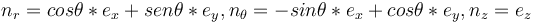.

Making the corresponding changes of coordinates and vectors in: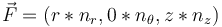.

The normal vector to the surface expressed in cylindrical coordinates: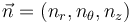,

so the scalar product of the function by the normal vector is: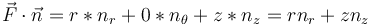.

Returning to the formulation of the DT and writing the previous results: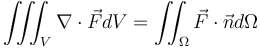,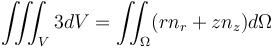,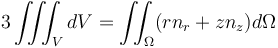,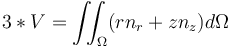,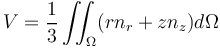,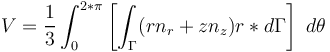,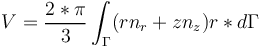,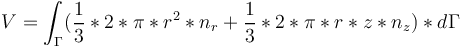,

So, as you can see in the last equation, in the line integral there is an initial term and in the second term (which is exactly the one you proposed) there is a 1/3 multiplicand.

Going over the expression and discussing it with theProfessor who gave me this function, since there is an nr component, it is possible that the calculation of the volume is a little more precise. I have performed the operations in COMSOL with the two expressions and they differ somewhat, not much, but somewhat.

I would like to know your opinion Jeff! :)

On the other hand, the note you left me: 2/ I checked off the "compute volume integral" checkbox to take manual control of the axisymmetric integratio" I don't quite understand, where does this option appear in COMSOL?

Thank you very much for your help,

Kind Regards,

Andres

Posted: 1 week ago

The same idea using the divergence theorem has been used here:

Thank you very much Henrik!

:)

Best, Andres

Posted: 1 week ago
Updated: 1 week ago

Hi Andres,

You could also use

F=r*er

and then the volume integral on the l.h.s. of the DT is equal to

2*V

while the line integral looks like the initial term in your version of it (with a multiplicant). All of those approaches are essentially equivalent. Since things are axisymmetric in your work, I preferred not to introduce an F that breaks that axisymmetry. Your derivation will be easier to extend to non axisymmetric cases for other readers of this Discussion Forum, so thank you for taking the time to type it out.

As far as accuracy goes, I wouldn't expect that one F will intrinsically yield more accurate results than another. The integrals are computed via Gaussian quadrature so they are not exact, and which one is closest to the exact value will likely depend on the shape of the hole, the integration order, and the mesh.

Regarding my comment "I checked off the "Compute volume integral" check box to take manual control of the axisymmetric integration": It's a typo on my part - I meant to type "I checked off the "Compute surface integral" check box to take manual control of the axisymmetric integration", see attached screenshot and Reference Manual, version 6.1, page 411. My apologies.

Jeff

-------------------
Jeff Hiller

Posted: 1 week ago

To calculate the volume of a hole inside an axisymmetric 2D domain in COMSOL Multiphysics, you can use the "Integral Evaluation" feature. Here's an overview of the process:

Create a 2D axisymmetric model in the COMSOL Model Builder, and define the geometry of the domain, including the hole.

Once the model is solved, go to the "Results" tab and select the "Integral Evaluation" feature.

In the "Integral Evaluation" settings, select "Volume" as the quantity to integrate over.

Under "Geometry," select the hole as the region to integrate over.

Click "Evaluate" to calculate the volume of the hole.

Additionally, you can also use the "Integral Evaluation" feature to calculate other integrals such as surface area, flux, and more.

Posted: 1 week ago

Suganth,

The point of this thread is that Andres does not want to include the hole in the geometry .

Jeff

-------------------
Jeff Hiller

Posted: 1 week ago

Hi,

actually the hole is in the geometry because its boundaries are. With the analytical method it is not in the mesh and this may save a little memory. Is there any other advantage of the method, is it more accurate? I see that it is quite elegant. I am just asking out of curiousity.

Cheers Edgar

-------------------
Edgar J. Kaiser
emPhys Physical Technology
www.emphys.com

Posted: 6 days ago

Hello Edgar,

We can have a philosophical debate as to whether the hole is in the geometry or not when only its boundaries are. I think we understand each other though.

The solution that I provided does not require meshing the interior of the hole; this is, as I understand it, what Andres was looking for.

Meshing the hole has a numerical cost. Usually it's a pretty low cost, in the grand scheme of things, and I wouldn't fret over it. For some odd-shaped holes, meshing the hole may be challenging while meshing the material around the hole is not. Again, that's a rather rare situation, and not really worth discussing at length. What I would say on the other hand is that if the volume of the hole changes over the course of a transient simulation (and Andres mentioned at the start of the thread that he is dealing with creep, so it is likely the case for him), then you have to solve an extra equation to deform the mesh in the interior of the hole if you are going to compute its volume without using the Divergence Theorem. This can have a more significant impact on how much time and memory is needed by the simulation.

Best,

Jeff

-------------------
Jeff Hiller

Posted: 6 days ago

Hi Jeff,

thanks for elaborating on this. It is definitely an interesting approach and I will bookmark the thread as a highlight.

Regarding the philosophy of holes. I am sometimes wondering whether the holes in a swiss cheese (Emmental) are part of the cheese or not. In case they were missing I would probably not accept the product to be genuine.

Cheers, Edgar

-------------------
Edgar J. Kaiser
emPhys Physical Technology
www.emphys.com

Posted: 6 days ago

Well, we have a blog post for you!

Jeff

-------------------
Jeff Hiller

Posted: 6 days ago

-------------------
Edgar J. Kaiser
emPhys Physical Technology
www.emphys.com

Posted: 4 days ago

Wow! This thread has more replies than an Elon Musk tweet ;-)

I think the conversation is very interesting and enriching for all of us who are "in love" with COMSOL :-)

I respond to forum participants who have quoted me at some point:

• Suganth, yes, Jeff is right. I was looking for a way to calculate the volume of the hole in a 2D-axisymmetric body without having to mesh or define an internal volume to the hole.

• Jeff, yes, you're right. The way of defining the vector function F does not affect the result in DT. I have used the function that you propose and the one that I indicated to you and the numerical result only varies slightly. If you do the development by hand with some simple body the analytical result is the same.

• Edgar, the truth is that I have not verified if the method by means of the DT or by means of a mesh of a volume is more precise. But meshing a volume that I am not going to use makes me a bit uneasy in case when doing calculations in very extreme situations that mesh deforms a lot and can give me problems. I am calmer with a method that does not require that auxiliary mesh. Thanks in any case :-)

• Thanks also to Henrik and Robert for participating in this discussion.

By the way, I think the Swiss cheese example is great :-), congratulations to the COMSOL team and thank you all again for your help.

Andres